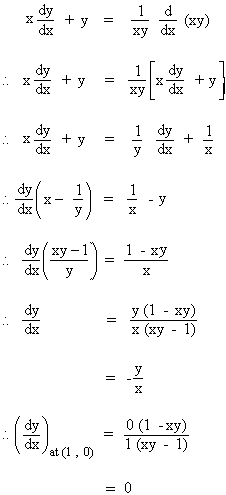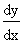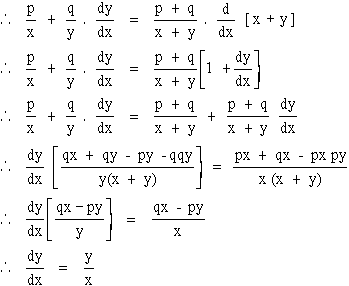Home MonkeyNotes Printable Notes Digital Library Study Guides Study Smart Parents Tips College Planning Test Prep Fun Zone Help / FAQ How to Cite New Title Request

Example 37

Find y at the point (1, 0) if xy = log (xy)

Solution :  xy = log (xy). Differentiating w. r. to. xYour browser does not support the IFRAME tag.

Example 38

If xp yp = ( x + y)p+q FindSolution :  xp yp = ( x + y)p+q

Note that here we will use the technique of logarithmic differentiation. i.e. Taking logs of both the sides, we get

p log x + q log y = (p + q) log (x + y)

Differentiating w. r. to x. we getIndex

4. 1 Derivability At A Point
4. 2 Derivability In An Interval
4. 3 Derivability And Continuity Of A Function At A Point
4. 4 Some Counter Examples
4. 5 Interpretation Of Derivatives
4. 6 Theorems On Derivatives (differentiation Rules)
4. 7 Derivatives Of Standard Functions
4. 8 Derivative Of A Composite Function
4. 9 Differentiation Of Implicit Functions
4.10 Derivative Of An Inverse Function
4.11 Derivatives Of Inverse Trigonometric Functions
4.12 Derivatives Of Exponential & Logarithmic Functions
4.13 Logarithmic Differentiation
4.14 Derivatives Of Functions In Parametric Form
4.15 Higher order Derivatives

Chapter 5